#Quality Digest

Featured Product
This Week in Quality Digest Live
CMSC Features
Etienne Nichols
What’s the difference?
Atul Minocha
Ryan E. Day
September Speaker Series in review
Ryan E. Day
Realigning a cornerstone of industry
David H. Parker
Practical implications for electronic distance measurement
CMSC News
Expands simulations for electrification and aerospace design
VSL hosts special edition of show at new center in Rotterdam
Latest line touts comprehensive coverage, ease of use
Blackfly S GigE line grows
Voting for 2022–2023 term will open at CMSC 2022 starting July 25
New facility in Toronto area will showcase multiple Hexagon product lines
API division named ‘Top External Provider 2018’
Exact Metrology selected for project
Faster and more powerful than ever beforeBio

CMSC

## Measurement Accuracy of a Mirrored Surface Using a Laser Tracker

Published: Friday, May 10, 2013 - 13:04

Laser trackers and the software that control them have revolutionized the way metrology data has been taken over the last 20 years. With software and hardware advances, taking measurements has become more efficient and accurate.

One of these advances in metrology software is the ability to measure the angle normal to a mirrored surface with a laser tracker by first measuring a stationary spherically mounted retroreflector (SMR) through the mirror, and then measuring the same SMR directly. This technique has become a replacement for the traditional method of measuring the angle normal to a mirrored surface with auto-collimating theodolites. With this improved measurement technique comes a question: What is the accuracy? There are two variables to this measurement technique that will be studied in this article to answer that question. The first is the distance between the laser tracker and the mirrored surface. The second is the angle of incidence of the laser beam to the mirrored surface.

The first set of data taken by the laser tracker will be of a fixed cube taken at fixed distances along a line. The cube will be fixed on a stable surface and SMR nests will be distributed around the cube and measured with the tracker. The laser tracker will be moved down a line at a fixed angle from the cube. Measurements will be taken at 10-foot intervals along this line. Before measurements are taken at each 10-foot interval, the laser tracker will be transformed to the common coordinate system stored in the stable SMR nests that were installed and measured at the beginning of the measurement process.

The second set of data taken by the tracker will be of a cube on a rotary stage while the tracker is stationary. The cube will be rotated 10° between measurement sets. A theodolite will be used to measure the angle of the cube. The cube angles measured by the laser tracker will be compared to the theodolite measurements and an accuracy analysis will be performed.

The goal of this study is to document the effect of distance and angle of incidence on mirrored surface measurement with a laser tracker.

### Laser tracker mirror measurement method

A mirrored surface can be measured with a laser tracker by measuring two points. The tracker is adjusted so that the laser beam is reflected off the surface of the mirror that is to be measured. Next, an SMR is placed on a stable stand so that it collimates the reflected laser beam. This is the first of the two required points. Next, the SMR is very carefully rotated so that it can be measured directly by the laser tracker. Great care should be taken to ensure that the SMR stand does not move. The direct measurement is the second required point. From these two points SpatialAnalyzer software, a product of New River Kinematics of Williamsburg, Virginia, is used to calculate the vector normal to the mirror and the position of the mirror plane. This method is explained graphically in figure 1.Figure 1: Construction of a plane from two laser tracker measurements

### Distance test

The distance measurement accuracy study was conducted in a 10K-class clean room on the Sunnyvale, California, Lockheed Martin campus. A granite surface plate at one end of the room was used to support the cube during the test. Tape was applied to the table and cube to act as a release agent. The cube was then bonded to the table with hot-melt glue.

The first test evaluates the effect of distance on the measurement of a mirrored surface. A laser tracker is used to measure a mirror cube at 10-foot intervals out to 90 feet for a total of nine stations.

A grid of 1.5-in. drift nests was bonded to an area of the floor that was approximately 65 ft × 36 ft. These nests were measured from 10 positions by a Leica 901 laser tracker, a Hexagon Metrology product. The United Spatial Metrology Network (USMN), a feature of SpatialAnalyzer, was used to optimize the uncertainty of the grid measurements. This grid of nests was used to transform the laser tracker into a common coordinate system as it is moved from station to station. See figure 2.

Next, a 3D theodolite system comprised of three theodolites was used to measure the cube and the drift nests. Two of the theodolites in the 3D system were auto-collimated on the cube and a point measured in SpatialAnalyzer. These measurements tied the cube position to the tracker nests in a common coordinate system. The theodolite network was bundled and a coordinate frame was created from the cube measurement. The cube frame is the working frame that the distance data are reported in. This will yield deviations of the tracker measurements with respect to the theodolite measurements of the mirror cube.

Uncertainty fields were created for the theodolite-measured points and the entire measurement set (trackers and theodolites) was optimized using the USMN with the point groups feature of SpatialAnalyzer.Figure 2: Distance test room layout

A line centered on the cube and extending to the corner of the clean room was laid out on the floor. This line was at approximately a 24° angle of incidence to the cube. In the SpatialAnalyzer job this line was called a “shoot line.” The angle of this line with respect to the cube was chosen for two reasons. First, the geometry of the room and cube position required a deviation from the cube normal to provide a 90-foot run. Second, 24° is not a very large angle of incidence and it wasn’t expected to affect measurement accuracy.

Laser tracker positions were marked on this line at 10-foot intervals out to 90 feet from the cube. The laser tracker was moved to each of these positions to measure the cube and grid of fixed nests. These measurements will yield cube-normal angles every 10 feet along the line that can be compared to the theodolite measurement of the cube-normal in the same coordinate frame to determine the tracker accuracy at distance.

An instrument stand was set up approximately 50 in. from the mirror cube and an SMR nest was installed. It was placed so that the reflected laser tracker beam from the mirror cube would collimate on the SMR installed in the nest. At each laser tracker position along the shoot line, 10 measurements were taken each of the direct SMR and the SMR reflected through the cube. The SMR was rotated between each direct and reflected measurement. This process was repeated for each station from 10 feet out to 90 feet from the cube in 10-foot intervals for a total of nine stations and 90 laser tracker data points total.

### Distance test results

The data were compiled and analyzed in a common coordinate frame that is based on the cube measurements. The first analysis was to compare the range of the minimum and maximum azimuth and elevation of the 10 measurements taken at each station. The range data yields the accuracy of the tracker in azimuth and elevation at each station. The data are reported in both decimal degrees and seconds of arc.Table 1: Range between min and max data points per station

The first plot (Table 1) is of the range between the minimum and maximum data points at each distance station. The increase of the range between the minimum and maximum values at distance is due to the tracker uncertainty increasing with distance.

From the plots of the data, the range between the minimum and maximum data points at each station jumps from 0.0013° (4.56 arc-sec) at 40 feet from the cube to 0.0047° (17.15 arc-sec) at 50 feet and continues to increase as the distance between the tracker and the cube increases.

Next, the maximum and minimum values of the 10 measurements taken at each station were plotted for both azimuth and elevation and reported in Table 2 and Table 3.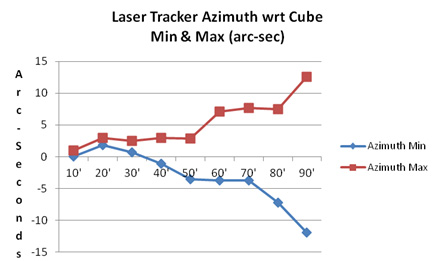Table 2: Min and max deviation, azimuthTable 3: Min and max deviation from 90°, elevation

Tables 2 and 3 show the deviation of the azimuth and elevation extremes at each station. These numbers diverge with distance due to the tracker accuracy. The minimum deviation from 90° at 80 feet in elevation was out-of-family with the deviations at 70 feet and 90 feet. Otherwise the data deviates with distance as expected.

Finally the average of the 10 measurements taken at each station was plotted for both azimuth and elevation in Tables 4 and 5 respectively.Table 4: Laser tracker average, azimuthTable 5: Laser tracker average, elevation

Averaging the 10 samples at each station yields much lower errors, especially at longer distance.

### Angle test

The second test in this study was to evaluate the effect of angle-of-incidence of a laser tracker’s laser beam with respect to a mirror cube while measuring the vector normal to the mirror cube. A machinist’s stage was secured to one end of a stable bench and the same Leica 901 tracker that was used for the distance test was set up at the opposite end. See figure 3. Next, a grid of 30 1.5 in. drift nests were bonded to the bench and measured by a Leica 901 laser tracker from five positions. The USMN feature of SpatialAnalyzer was used to optimize the uncertainty of the grid measurements. The machinist’s stage was used to rotate the mirror cube about the gravity vector in 10° increments. At each 10° increment the mirror cube was measured by both the laser tracker and 3D theodolite system with respect to the grid of points. Because the grid of points is common to both the tracker and theodolite data sets the two data sets can be compared and analyzed for accuracy.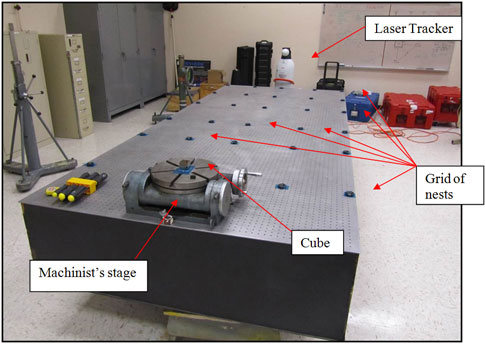Figure 3: Angle test layoutFigure 4: Laser tracker positions for USMN data set

Four Leica TM 5100A theodolites were set up around the bench. Two of the theodolites were auto-collimated on the cube and measured a point on the cube using SpatialAnalyzer. The grid of points was measured with all four theodolites and the network was bundled. The bundle yielded the coordinates of each drift nests and the mirror cube. A coordinate frame was created on the mirror cube and called the baseline cube position. As the cube was rotated the theodolites were positioned so that two were collimated on the cube and the remaining two were positioned to achieve acceptable apex angles for the 3D theodolite measurements. This approach ensured that each cube position was related back to the baseline cube position and the drift nests, which yielded the cube rotation relative to the baseline position as well as correlating the theodolite data with the laser tracker data. See figure 5 for the theodolite setup around the cube and optical bench and a 3D theodolite system.

Uncertainty fields were created for each theodolite data set. The laser tracker data of the drift nests was imported and the laser tracker was used to measure the grid of points. Using USMN with point groups in SpatialAnalyzer these data were networked and optimized. The laser tracker was used to measure the mirror cube using two points 10 times. This measurement plan was repeated at each cube rotation position for a total of eight cube positions and 80 tracker data points.Figure 5: Angle test measurement layout and 3D theodolite system

The USMN feature of SpatialAnalyzer was used to network the tracker data and the theodolite data into a single optimized network. The laser tracker beam was aimed at the mirror cube and an instrument stand was positioned so that an SMR in a nest mounted on the stand collimated the reflected laser beam. The laser tracker was used to measure the SMR 10 times, both reflected through the mirror and directly, alternating between direct and reflected measurements.

A line was created from one of the reflected measurements. The angle of this line with respect to the cube about the gravity vector was determined to be 9.9833° from the cube normal, therefore the first test was at 9.9833° from the cube normal. See figure 6. The test captured data from approximately 10° (9.9833° actual) to approximately 80° (79.9858° actual) in 10° increments. In order to simplify reporting these data, the increments were listed in even 10° increments. See Table 6 for the actual values.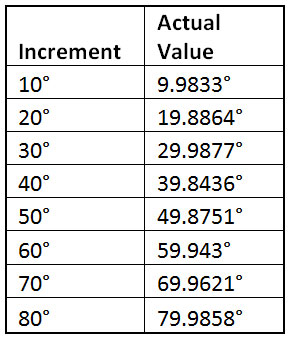Table 6: Angle test cube positionsFigure 6: Angle test laser tracker position

### Angle test results

Data were compiled and analyzed in a common coordinate frame that was based on the cube measurements. Data reported in the charts in this section are relative to the actual cube position as measured by the theodolite system by the laser tracker. These data show the deviation of tracker data from theodolite data.

The first analysis was to compare the range of the minimum and maximum azimuth and elevation of the 10 measurements taken at each station. The range data yield the accuracy of the tracker in azimuth and elevation. These data are reported in both decimal degrees and seconds of arc.Table 7: Laser tracker min to max range for azimuth and elevation

It can be seen that as the angle of incidence increases, the range from the minimum to maximum values also increases. The measurement uncertainty increases as the angle of incidence increases. Also note that the measurement at 10° had a higher error than at 20° through 50°. The small angle between the lines created by the reflected and direct measured points causes the angle calculation to be very sensitive. This yielded a higher uncertainty than angles of incidents that were greater than 10°.

Next, the maximum and minimum values of the 10 measurements taken at each station were plotted for both azimuth and elevation. These data are provided in both decimal degrees and seconds of arc.Table 8: Deviation of the azimuth and elevation extremes at each station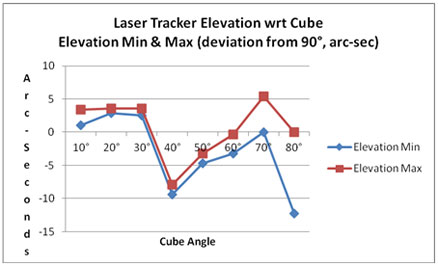Table 9: Deviation of the azimuth and elevation extremes at each station

The previous plots show the deviation of the azimuth and elevation extremes at each station. Again, these numbers diverge as the angle of incidence increases with an elevated error at 10°. The scale of the graphs causes the numbers to appear to diverge from the actual cube angle, but the displacements are relatively small. What is important is the displacement between the minimum and maximum value at each cube angle.

Finally the averages of the 10 measurements taken at each station were plotted for both azimuth and elevation. These data are provided in both decimal degrees and seconds of arc.Table 10: Laser tracker average, azimuthTable 11: Laser tracker average, elevation

Averaging these data yield azimuths from +8 arc-seconds to -6 arc-seconds across the 70° range in azimuth and +3 arc-seconds to -8 arc-seconds in elevation across the same range.

It should be noted that as the angle of incidence increased, the image of the laser beam on the cube distorted from a circular dot to a cigar-shaped smear. Figures 7 and 8 show the beam image at approximately 80°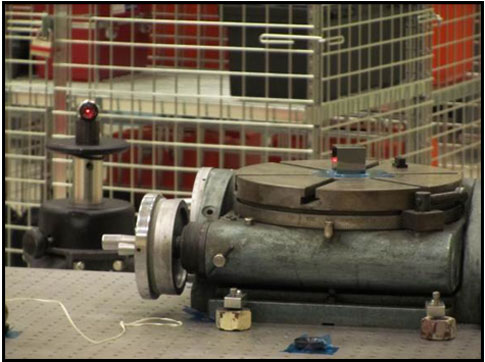Figure 7: Laser beam at 80°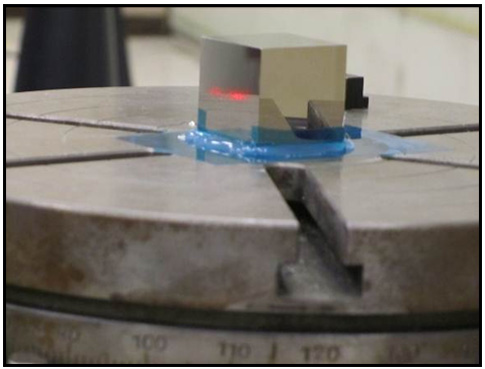Figure 8: Laser beam on cube at 80°

### Conclusion

The laser tracker distance test yielded data that show a range of +/-5 arc-seconds for data sets of 10 samples out to 50 feet. Beyond 50 feet, the uncertainty increases with distance. This error is due to the laser tracker uncertainty. It should be noted that the uncertainty dropped to +/-2.5 arc-seconds in azimuth and +8/-6 arc-seconds in elevation when data sets at each station were averaged.

At distances of 50 feet or less, a mirrored surface can be measured by a laser tracker with acceptable accuracy. Multiple mirror measurements (10 or more) should be taken at any distance to ensure that the SMR is stable. The SMR should be rotated between each direct and reflected shot to ensure stability. At distances greater than 80 feet, large data sets (20–50 mirror measurements) should be taken and averaged for acceptable uncertainty.

The laser tracker angle test showed that at 10°, the uncertainty was 10 arc-seconds in azimuth and 4 arc-seconds in elevation. At 20° the azimuth uncertainty dropped to -3 arc-seconds and elevation stayed the same. Past 30° the uncertainty oscillated between -5 arc-seconds and +12 arc-seconds for azimuth and -12 arc-seconds and +5 arc-seconds for elevation, increasing with angle. The instability of these measurements is believed to be due to the measurement method. In the distance test, the cube and the theodolites were stationary and only the measuring tracker moved. In the angle test the cube and theodolites moved while the tracker remained stable. For each station the theodolites required a completely new setup. These multiple setups could have caused the cube angles to change slightly which may cause data to appear to oscillate. Fortunately data extremes do fall within levels that are acceptable for many measurements.

It should be noted that the tracker was able to measure the cube when the angle of incidence was 80° and return data with lower than expected uncertainty.

When measuring mirror cubes at varying angle of incidence from the laser tracker, multiple data points should be taken and averaged, especially when the angle of incidence is greater than 50°

At an angle of incidence below 50°, a mirrored surface can be measured with acceptable accuracy. It should be noted that at 10° or less the uncertainty is slightly higher but still fewer than 3 arc-seconds. Multiple mirror measurements (10 or more) should be taken at any distance to ensure that the SMR is stable. The SMR should be rotated between each direct and reflected shot to ensure stability. At an angle of incidence above 50° the uncertainty increases with increased angle. At angles of incidence greater than 50° large data sets (20–50 mirror measurements) should be taken and averaged for acceptable uncertainty.

### References

1. SpatialAnalyzer is a product of New River Kinematics of Williamsburg, VA
2. The Leica 901 laser tracker is a product of Hexagon Metrology
3. SpatialAnalyzer and USMN are products of New River Kinematics of Williamsburg, Virginia.

### Discuss